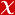## Energy dependence and fluctuations of anisotropic flow in Pb-Pb collisions at root(NN)-N-s=5.02 and 2.76 TeV

JOURNAL OF HIGH ENERGY PHYSICS, vol.2018, no.7, 2018 (SCI-Expanded)• Publication Type: Article / Article
• Volume: 2018 Issue: 7
• Publication Date: 2018
• Doi Number: 10.1007/jhep07(2018)103
• Journal Name: JOURNAL OF HIGH ENERGY PHYSICS
• Journal Indexes: Science Citation Index Expanded (SCI-EXPANDED), Scopus
• Keywords: Heavy Ion Experiments, RELATIVISTIC NUCLEAR COLLISIONS, ELLIPTIC FLOW, AZIMUTHAL ANISOTROPY, TRANSVERSE-MOMENTUM
• Bursa Uludag University Affiliated: No

#### Abstract

Measurements of anisotropic flow coefficients with two-and multi-particle cumulants for inclusive charged particles in Pb{Pb collisions at root s(NN) = 5 : 02 and 2.76TeV are reported in the pseudorapidity range vertical bar eta vertical bar < 0 : 8 and transverse momentum 0 : 2 < p(T) < 50 GeV/c. The full data sample collected by the ALICE detector in 2015 (2010), corresponding to an integrated luminosity of 12.7 (2.0) mu b(-1) in the centrality range 0{80%, is analysed. Flow coefficients up to the sixth flow harmonic (v6) are reported and a detailed comparison among results at the two energies is carried out. The pT dependence of anisotropic flow coefficients and its evolution with respect to centrality and harmonic number n are investigated. An approximate power-law scaling of the form v(n) (p(T)) similar to p(T)(n/3) is observed for all flow harmonics at low p(T) (0.2 < p(T) < 3 GeV/c). At the same time, the ratios v(n)/v(m)(n/m) are observed to be essentially independent of pT for most centralities up to about pT = 10 GeV/c. Analysing the di ff erences among higher-order cumulants of elliptic flow (v(2)), which have di ff erent sensitivities to flow fluctuations, a measurement of the standardised skewness of the event-by-event v(2) distribution P (v(2)) is reported and constraints on its higher moments are provided. The Elliptic Power distribution is used to parametrise P (v(2)), extracting its parameters from fi ts to cumulants. The measurements are compared to di ff erent model predictions in order to discriminate among initial-state models and to constrain the temperature dependence of the shear viscosity to entropy-density ratio.